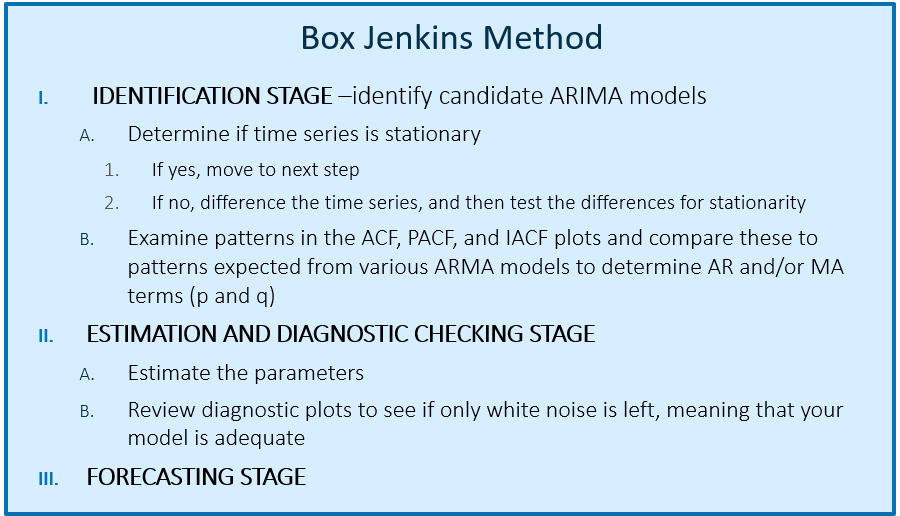## SAS Visual Forecasting 8.4: Interpreting Results and Diagnostic Plots

Started ‎08-14-2019 by
Modified ‎08-14-2019 by
Views 5,922

Using the Visual Forecasting 8.4 visual interface (the pipeline interface, i.e., Model Studio) you will get summary results including graphs, as shown below. Using Visual Forecasting 8.4’s coding interface (SAS Studio V), you can get diagnostic plots, such as ACF, PACF, IACF and white noise plots.

All of these plots are very similar to what you get with Forecast Server. If you already know how to interpret Forecast Server graphs, you can stop reading now. Otherwise, keep reading.

## VF 8.4 Visual Interface: Results Graphs

In our initial example, we use the standard sashelp.pricedata data set, with the data settings as follows: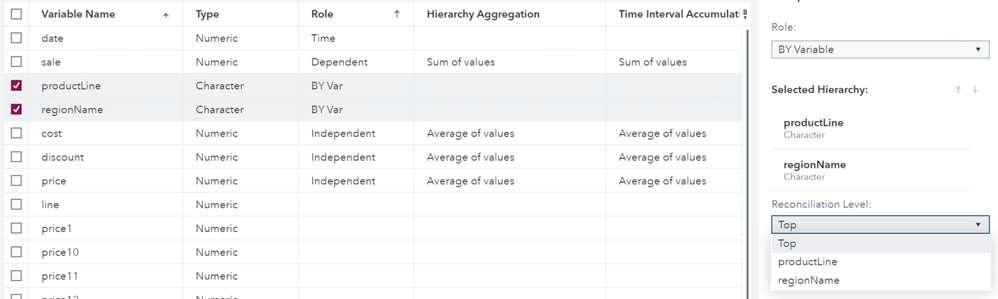Select any image to see a larger version.
Mobile users: To view the images, select the "Full" version at the bottom of the page.

and the Auto-forecasting node Model Generation settings and Model Selection settings as shown below: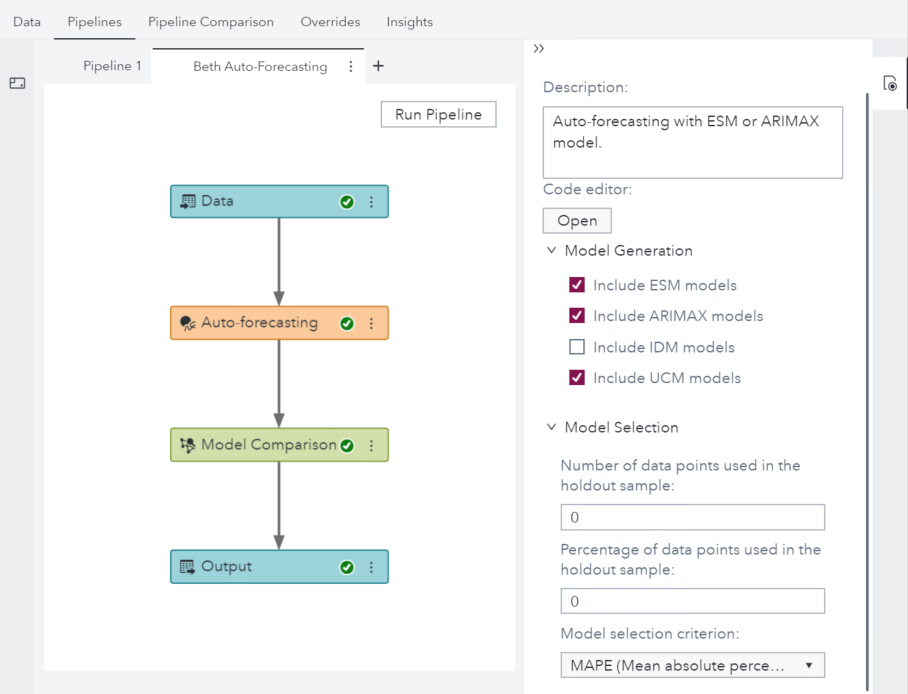We run the Auto-forecasting template and view the results by right-clicking the Auto-forecasting node and selecting Results.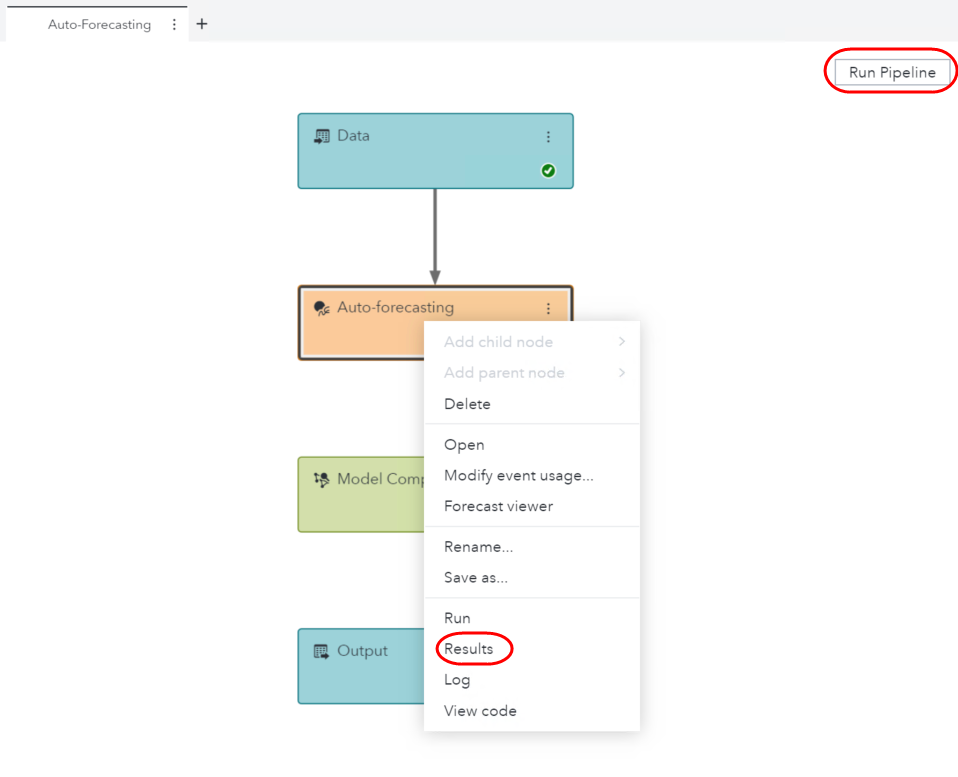We see in the Results that we have a total of 5 series.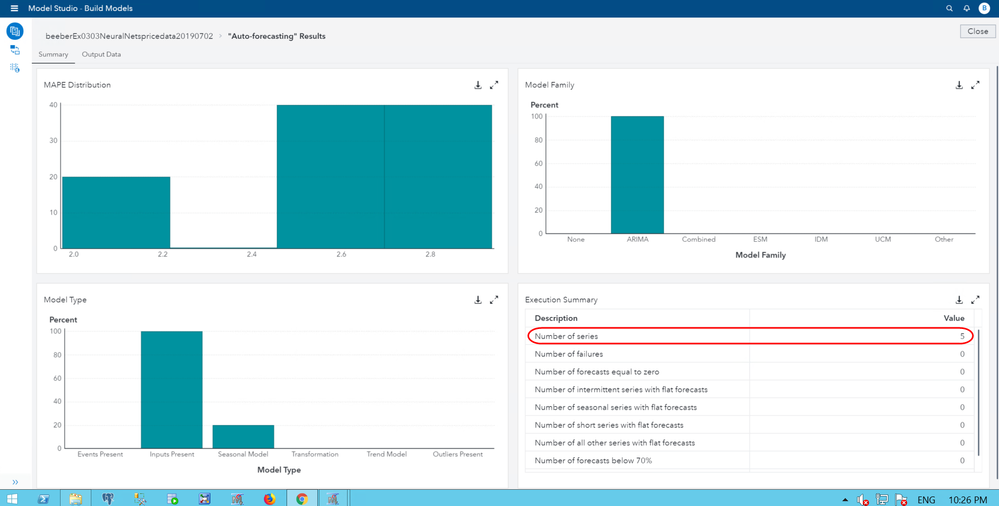### MAPE Distribution

The MAPE Distribution graph is a histogram. It shows on the Y axis (vertical axis) what percent of your time series had a MAPE within a certain range. You can roll over the bars to get the average MAPE and the percent of series that fall in that bin. Below we see that 20 percent of the series (i.e., 1 of our 5 series) falls into the first bar, with an average Mean Absolute Percent Error of 2.0953.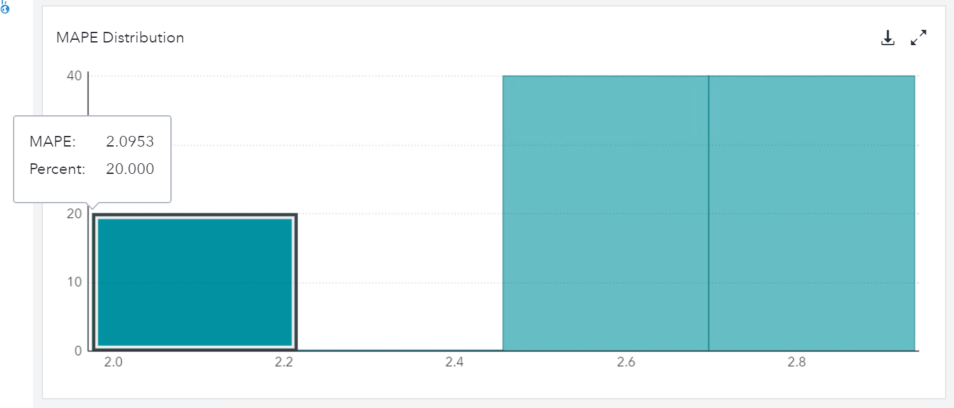MAPE measures the accuracy of your forecasting model as a percentage. This makes it much easier to interpret than root mean square error, which has a magnitude related to the magnitude of the data values.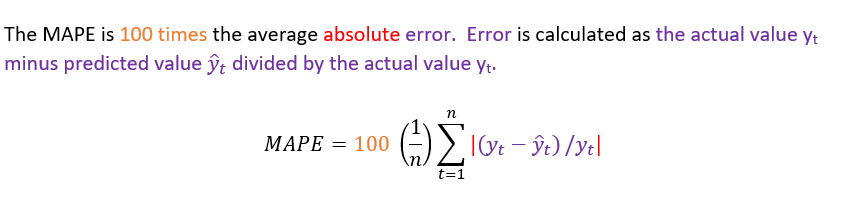Acceptable MAPEs will vary by situation and domain, but in many situations a MAPE less than 5 could be considered quite good.

### Model Family

The Model Family may be:

• None
• AutoRegressive Integrated Moving Average (ARIMA)
• Combined
• Exponential Smoothing Model (ESM)
• Intermittent Demand Model (IDM)
• Unobserved Components Model (UCM)
• Other

In this example, 100 percent (all 5) of the time series were modeled using ARIMA models.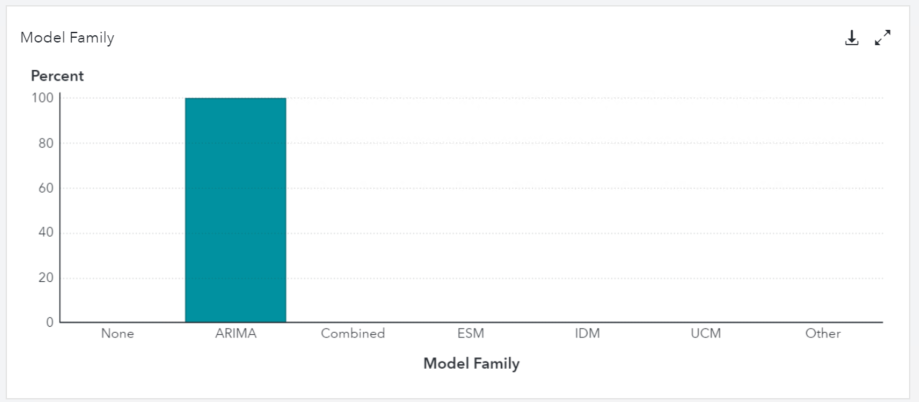### Model Type

The Model Type histogram tells us what percentage of the series modeled included events, inputs, seasonality, transformations, trends, or outliers. Below we see that all of the models used inputs (exogenous factors, i.e., independent variables). One of the models (20 percent of our 5 models) exhibited seasonality so a seasonal term was used.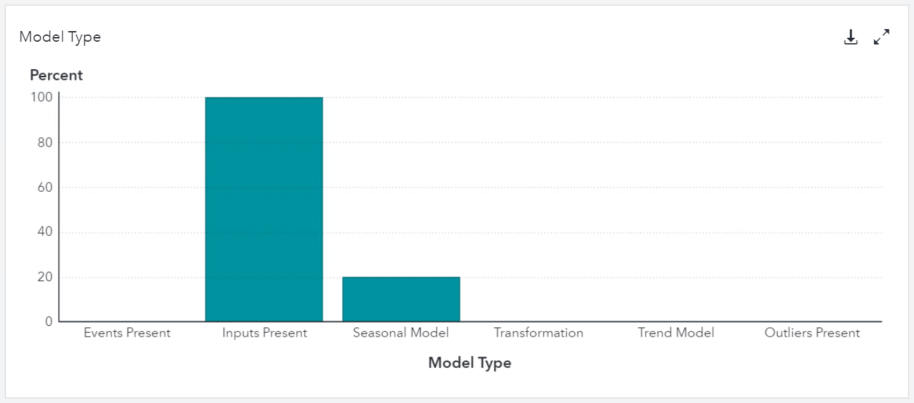### Execution Summary

The execution summary gives us information on how many series failed (e.g., model did not converge), the number of seasonal series with flat forecasts, and so on.## VF 8.4 SAS Studio Interface: Diagnostic Plots

The SAS Visual Forecasting 8.4 visual interface does not show us diagnostic plots, but we can use Visual Forecasting 8.4’s coding interface (SAS Studio V) to create diagnostic plots, such as:

• ACF plot
• PACF plot
• IACF plot
• White Noise plot

Below I use the SAS Studio V task under SAS Viya Forecasting called Time Series Exploration to create PROC TSMODEL code. PROC TSMODEL is the main procedure for time series analysis.

Note that we are using the task under SAS Viya Forecasting not the task with the same name under SAS Forecasting!PROC TSMODEL itself does not create diagnostic plots, but it does create the output we need to draw our own plots.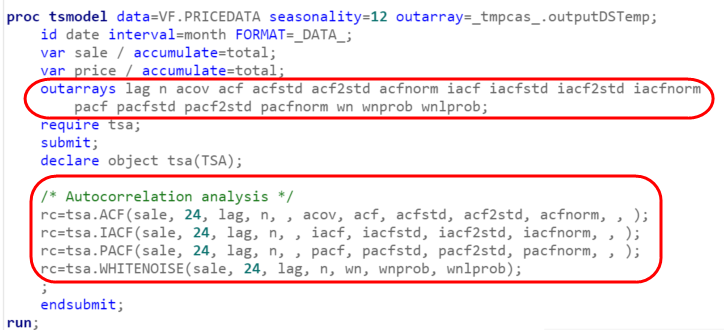We will draw the plots using PROC SGRENDER as shown, still using the Time Series Exploration task under SAS Viya Forecasting. We now move to the Analysis tab, and check the diagnostic plots we want to see.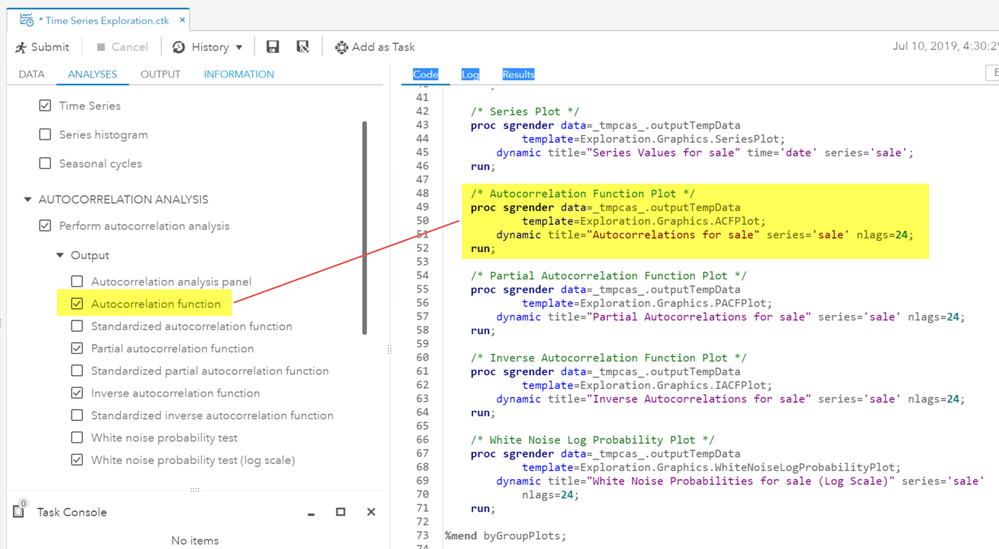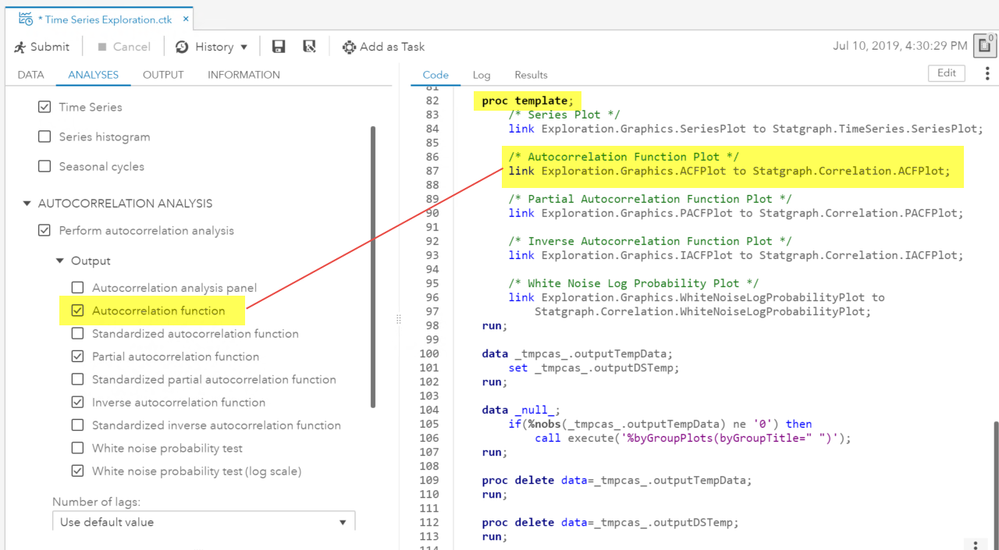PROC SGRENDER creates plots from templates that are created with the Graph Template Language (GTL). To learn more about PROC SGRENDER see the documentation.

### ARIMAs

Let’s remind ourselves what an ARIMA model is. Recall that AR terms (p) are lags of the observations. Recall that MA terms (q) are lags on the error terms.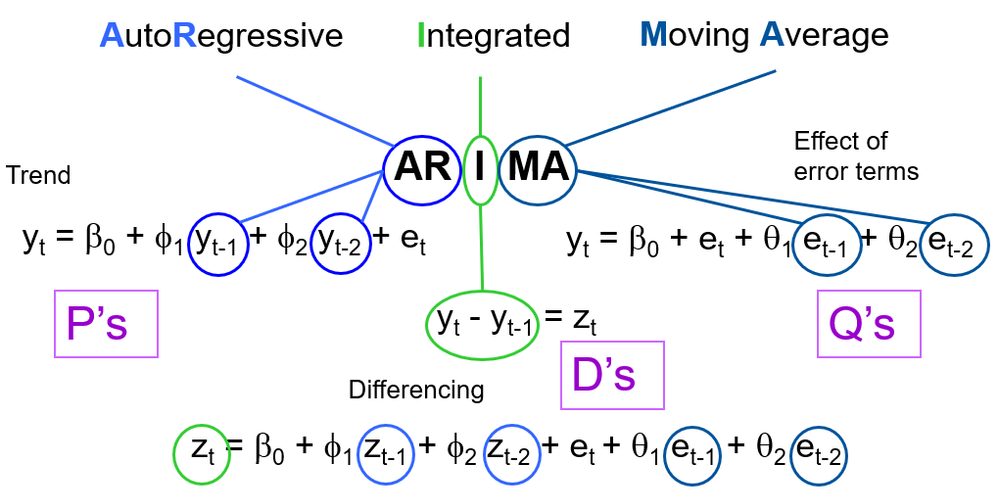Graphic courtesy of Joe Katz and Anthony Waclawski

## Diagnostic Plots

Interpreting diagnostic plots is more of an art than a science. To do it well, I recommend that you consider all of your diagnostic plots together, examine multiple examples, and gain experience yourself trying different p, d, q terms. For a more detailed understanding, explore the resources listed in the references section below. This article provides just a couple of textbook examples and few general rules of thumb.

We will consider four types of diagnostic plots:

• Autocorrelation Function (ACF)
• Partial Autocorrelation Function (PACF)
• Inverse Autocorrelation Function (IACF)
• White Noise

They can all be used:

• BEFORE MODELING to view autocorrelation of the original time series data and consider appropriate models to capture that autocorrelation, or
• AFTER MODELING, to see if autocorrelation still exists in the model residuals, which would mean you need to try a different model.

As a first step, let’s look at an example autocorrelation plot to orient ourselves to the graph. Again we use the pricedata data set, where sale is the dependent variable that we are trying to forecast.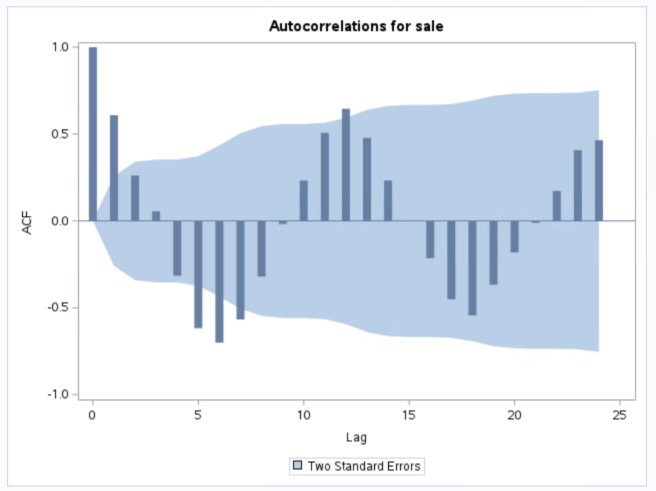Look at the first bar, where the lag = 0. This bar will always equal 1.0, that is, an observation yt is always perfectly correlated with itself.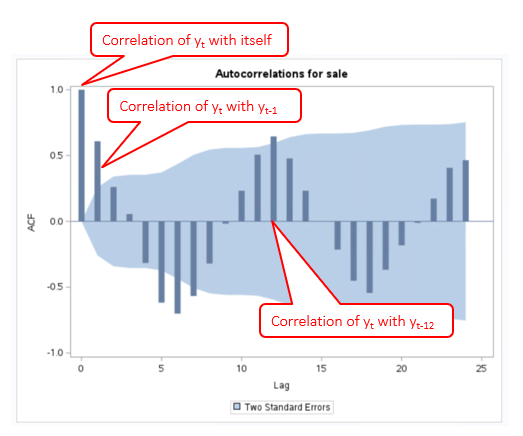Then we look at the second bar, and see that the correlation of the current observation yt is about 0.6 with the observation one period (in our case one month) in the past (yt-1). This bar extends outside the two standard error shaded blue area, which leads us to believe that it is significant. A spike at lag 1 for our sales data means that December’s sales are correlated with November’s, November’s sales are correlated with October’s, and so on. This ACF(1) is called first-order autocorrelation and may be referred to as serial correlation.

We also see some inverse correlations at lags 5, 6, 7. This indicates that December sales may be negatively correlated with May, June and July sales.

The final significant positive correlation lag we see is at lag 12. That means that this December’s sales are likely correlated with last December’s sales. This also makes sense if we know that sales tend to have annual cycles.

Always consider sensible lags at spikes (such as 12 for monthly data), 4 for data that may exhibit quarterly cycles, 24 for hourly data, and so on. Use your domain knowledge and common sense in conjunction with the autocorrelation plots to try to develop the best forecasting model.

### Autocorrelation Function (ACF)

The autocorrelation plots help you determine whether a time series is stationary or nonstationary. For example, if the ACF decreases rapidly, it indicates that the time series is stationary. In contrast, if the ACF plot decays very slowly, that indicates that the series is nonstationary.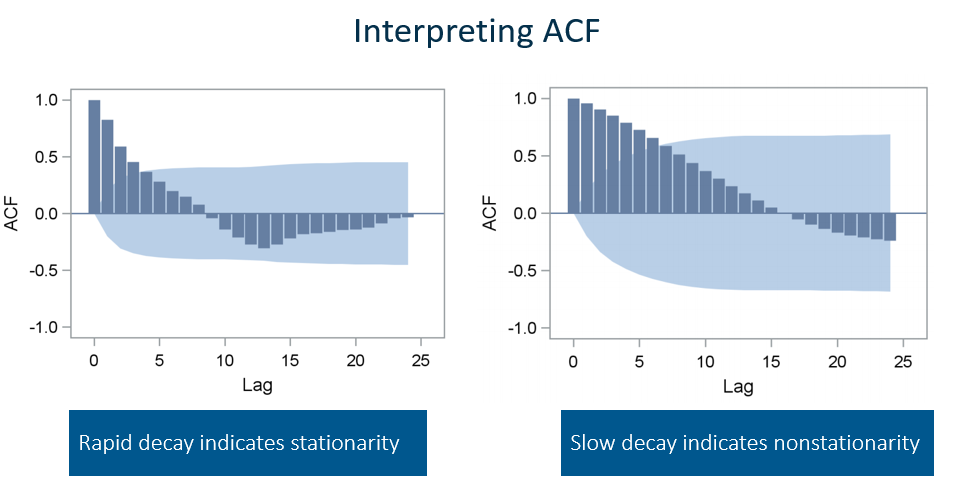REMINDER: A stationary time series is one whose statistical properties (e.g., mean, variance, autocorrelation) are constant over time. Time series with either trends (increasing or decreasing over time) or seasonality are not stationary.

If you have significant spikes in the ACF, there is systematic variation that can be extracted from the series, and it’s time to get busy building or modifying your forecasting model. If you have no significant lags in the ACF (i.e., no spikes outside the blue shaded area shown above), then the series is white noise, and a model will not help you extract useful information.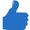RULE OF THUMB: The ACF helps you diagnose MA terms, and can support your diagnosis of a need for AR terms. If ACF bars drop off after 0, then try MA(1) model.

### Partial Autocorrelation Function (PACF)

The PACF adjusts for all previous lags, in contrast to the ACF which does not adjust for other lags. So, for example, the PACF at lag 5 is the correlation that results after removing the effect of correlations due to the terms at lags 4, 3, 2, and 1.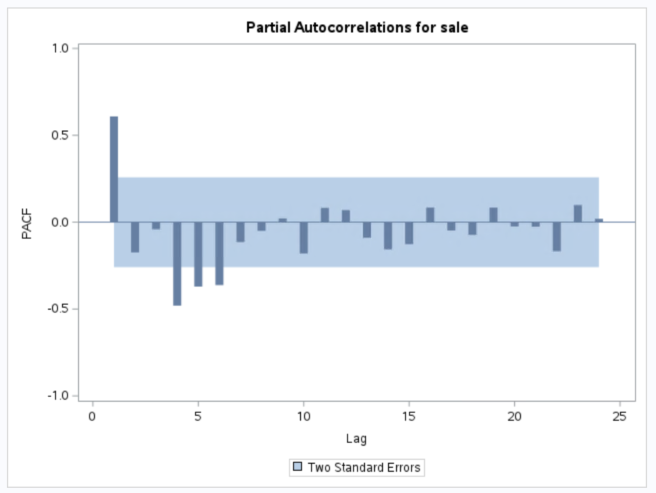RULE OF THUMB: Use PACF to help select AR terms. If PACF bars drop off after 1 then try AR(1) model. PACF spikes indicate lags to try for a pure AR model.

### Inverse Autocorrelation Function (IACF)

The IACF also helps you diagnose AR and MA terms. The IACF commonly suggests subset and seasonal autoregressive models better than the PACF, and it can be useful for detecting over-differencing. If the data have been over-differenced, the IACF looks like an ACF from a nonstationary process (that is, it exhibits slow decay, as shown for the right hand side ACF two graphs up).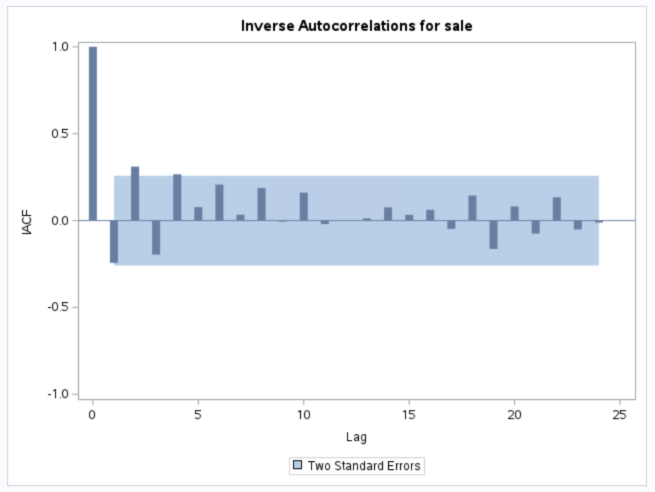RULE OF THUMB: If the IACF dies off exponentially, it suggests an MA(1), i.e., you should try setting q=1.

### White Noise Test

White noise is a purely random process. If your original time series data turns out to be white noise, there is no information to be gained by fitting a model. Just close your computer and go home.

If the series is not white noise, you can try an autocorrelation model, such as AR(1), an MA model, such as MA(1), or a low-order mixed ARMA model. You can also use the ACF, PACF, and IACF plots as described above to help you decide what model to try first.

Once you have fit the model of your choice, test the model residuals to see if they are white noise. If the residuals are white noise, you have a reasonable model. If the residuals are not white noise, then you can probably get additional information from a more complex model.

SAS Visual Forecasting generally uses a chi-square statistic (Ljung-Box formula shown below) to determine if the residuals are white noise.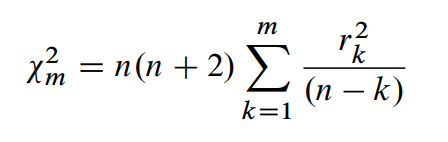where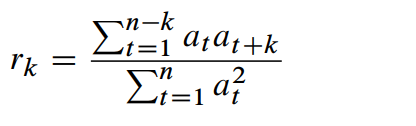and at is the series being evaluated.

You decide if you how to set your type 1 error (a) rate; 0.01 or 0.05 are commonly used. Let’s say you select 0.01. A p-value less than 0.01 suggests that the residuals are not white noise, meaning that your model was inadequate.

If the p-value is greater than 0.01, the white noise test plots show that you cannot reject the null hypothesis that the residuals are not correlated. Ok that last sentence has a lot of negatives, but that’s just how old school statisticians like me speak. Nothing is ever accepted, it’s all about failing to reject. But in short, if the p-value is greater than 0.01 you conclude that your residuals are correlated and are not white noise.

Put a different way, the White Noise Test tests that none of the autocorrelations of the series up to a given lag are significantly different from 0. If none of the autocorrelations of the series are significantly different from 0, that indicates that no ARIMA model is needed for the series (because there is no information in the series to model). If the series is nonstationary, we expect the white noise hypothesis to be rejected, i.e., suggesting that some of the autocorrelations may be significantly different from 0.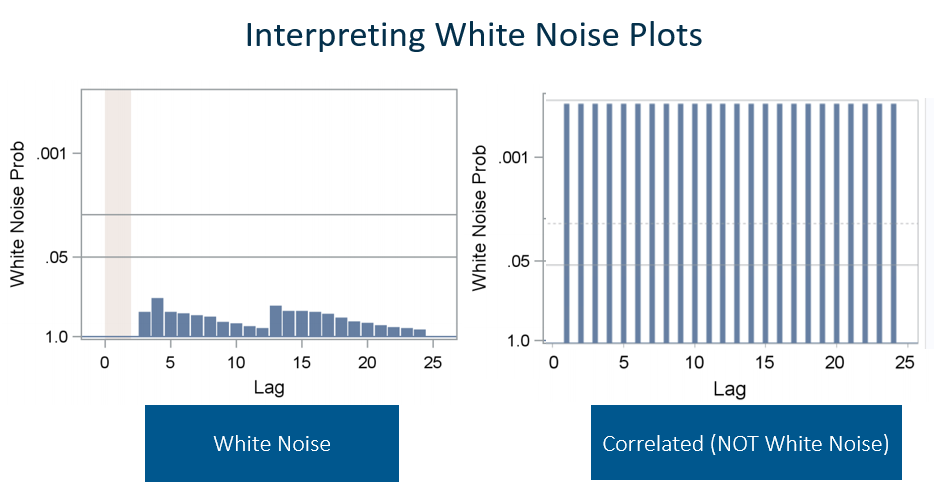If the series is nonstationary, we can transform it to a stationary series by differencing. Differencing means that instead of modeling the sales values themselves, we model the difference from one period to the next.

First order differencing computes the difference between consecutive observations. First order differencing is commonly used to make a nonstationary time series stationary if there is an upward or downward trend in the data. Other orders of differencing can also be useful. For example, seasonal differencing for monthly data that exhibits seasonality, computes the difference between an observation and the observation 12 time periods ago. For example, subtract January 2018 from January 2019, and so on.

PRO TIP: Don’t base your decision on a single plot. Use all of the plots along with your knowledge and understanding of the data and the domain to make the best informed decision. And don’t hesitate to try different models! SAS Visual Forecasting makes it quick and easy to adjust your p,d,q. You can also use automated forecasting via either the visual interface or using the ATSM package in SAS Studio to get you started with a decent model, that you can further tweak if you would like.

### Caveats

For ease of reading and following this article, I am not carefully noting that the ACF, PACF, and IACF shown here are actually the sample ACF, sample PACF, and sample IACF, not the population ACF, PACF, and IACF. Because they are sample they only estimate the actual population and thus, we do not consider them significant if within the 2 standard deviations.

Also, remember, when you use a 95% confidence interval, you expect that 5% of your results will be spurious. That is, one in 20 of the bars in your plots is expected to be outside the 95% confidence interval strictly by chance, not because it is significant.

Most textbooks on time series analysis, such as Pankratz (1983), discuss the theoretical autocorrelation functions for different kinds of ARMA models. A few resources are listed at the end of this article if you wish to continue to expand your understanding of forecasting methods.

## Summary

Diagnostic plots can be very helpful in determining the best model for your time series data, as well as determining if the model you selected was adequate. The three stages of ARIMA modeling as outlined by Box and Jenkins in 1976 and reiterated in the SAS documentation are shown below.